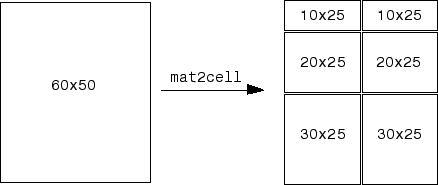Main Content

# mat2cell

Convert array to cell array whose cells contain subarrays

## Syntax

``C = mat2cell(A,dim1Dist,...,dimNDist)``
``C = mat2cell(A,rowDist)``

## Description

example

````C = mat2cell(A,dim1Dist,...,dimNDist)` divides array `A` into smaller arrays and returns them in cell array `C`. The vectors `dim1Dist,...dimNDist` specify how to divide the rows, the columns, and (when applicable) the higher dimensions of `A`. The smaller arrays in `C` can have different sizes. `A` can have any data type.```

example

````C = mat2cell(A,rowDist)` divides array `A` into an `n`-by-1 cell array `C`, where `n` equals the number of elements in `rowDist`.```

## Examples

collapse all

Create a 5-by-4 numeric array.

`A = reshape(1:20,5,4)'`
```A = 4×5 1 2 3 4 5 6 7 8 9 10 11 12 13 14 15 16 17 18 19 20 ```

Divide `A` into two 2-by-3 and two 2-by-2 subarrays. Return the subarrays in a cell array.

`C = mat2cell(A,[2 2],[3 2])`
```C=2×2 cell array {2x3 double} {2x2 double} {2x3 double} {2x2 double} ```

Display the subarrays in `C` using the `celldisp` function.

`celldisp(C)`
``` C{1,1} = 1 2 3 6 7 8 C{2,1} = 11 12 13 16 17 18 C{1,2} = 4 5 9 10 C{2,2} = 14 15 19 20 ```

Create an array.

`A = reshape(1:20,5,4)'`
```A = 4×5 1 2 3 4 5 6 7 8 9 10 11 12 13 14 15 16 17 18 19 20 ```

Divide the rows of `A` so that the cell array contains two subarrays. Since the first element of `rowDist` is `1`, the first cell of `C` contains the first row of `A`. The second element of `rowDist` is `3`, so the next cell of `C` contains the next three rows of `A`. The sum of the elements of `rowDist` equals the number of rows of `A`.

```rowDist = [1 3]; C = mat2cell(A,rowDist)```
```C=2×1 cell array {[1 2 3 4 5]} {3x5 double } ```

Display the subarrays.

`celldisp(C)`
``` C{1} = 1 2 3 4 5 C{2} = 6 7 8 9 10 11 12 13 14 15 16 17 18 19 20 ```

## Input Arguments

collapse all

Input array.

Vectors describing the distributions of input array elements along each dimension, specified as numeric vectors.

For example, if `A` is a 60-by-50 array, then you can specify this argument as `[10 20 30],[25 25]` to divide `A` as shown in the code and figure. `C` is a cell array that contains the six subarrays split out of `A`.

```C = mat2cell(A,[10 20 30],[25 25]) ```For the `K`th dimension of `A`, specify the elements of the corresponding vector `dimKDist` so that `sum(dimKDist)` equals the size of the `K`th dimension.

If the `K`th dimension of `A` has a size of zero, then specify the corresponding vector `dimKDist` as the empty array, `[]`, as shown in the code.

```A = rand(3,0,4); C = mat2cell(A,[1 2],[],[2 1 1]); ```

Vector describing the distribution by rows of the input array, specified as a numeric vector. When you do not specify how to divide `A` along any other dimension, the `mat2cell` function returns an `n`-by-1 cell array `C`, where `n` equals the number of elements in `rowDist`.

Each element of `rowDist` specifies the number of rows in the subarray that is in the corresponding cell of `C`. The sum of the elements of `rowDist` must equal the number of rows of `A`.

## See Also

### Topics

Introduced before R2006a

Download ebook